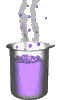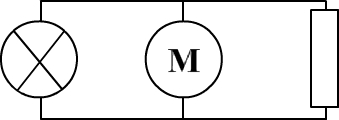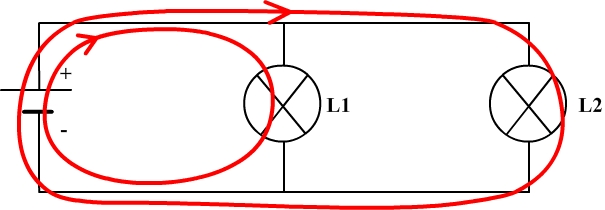Science class online
Chemistry and physics lessons

# Electricity

Free online electricity lessons for elementary school,  middle school and high school.Science class Chemistry Electricity Optics Mechanics Electricity lessons Electrical components -Two-terminal electronic components - Basic electrical components - Diodes - What's a resistor ? - How to determine a resistor value ? - Resistors effects in circuits - How to use a resistor ? - Characteristic curve of a resistor How to build simple circuits and draw diagrams - How to build a basic electrical circuit - How to draw diagrams of electric circuits The electric current - Conductors and insulators - Direction of electric current in a circuit - The dangers of electricity - Current intensity - units how to measure current ? The Voltage - The voltage and its units - How to measure a voltage ? - Voltage in open and closed circuits - Rated current and voltage for a lamp Alternating voltage and current - Alternating currents effects on led - What are alternating current and voltage ? - Periodic alternating voltage and its properties - Oscilloscope - Oscillogram - Frequency - Measuring RMS voltage with a voltmeter Serie circuits - What's a serie circuit ? - Series circuit properties - Short circuit in  series circuit - Current law in series - circuits - Voltage law in series circuits Parallel circuits - What's a parallel circuit ? - Some parallel circuits properties - Short circuits in parallel circuits - Nodes and branches in parallel circuits - Current laws in parallel circuits - Voltage laws in parallel circuits Laws of electricity - Ohm's law - Current laws in series circuits - Voltage laws in series circuits - Current laws in parallel circuits - Voltage laws in parallel circuits Generating electricity - Voltage for coil wires What is an alternator ? - Alternating currents and voltages - Generating electricity in power plants Electric power and energy - Electric power and power rating - Electric power consumption by an electrical device - Relationship between - Electric power and energy

 _____________________________________________________   _____________________________________________________

## Parallel circuits

### What is a parallel circuit ?

 If the two terminals of an electric component are directly connected to the two terminals of another component then these ones are said to be connected in parallel Example of parallel connectionThe motor and the bulb are connected in parallel. The motor and the resistor are connected in parallel. ______________________________________ ______________________________________ A circuit is said to be a parallel circuit if all its components are connected in parallel. This kind of circuit always includes at least two current loops. Example of parallel circuit and its current loops____________________________________

____________________________________